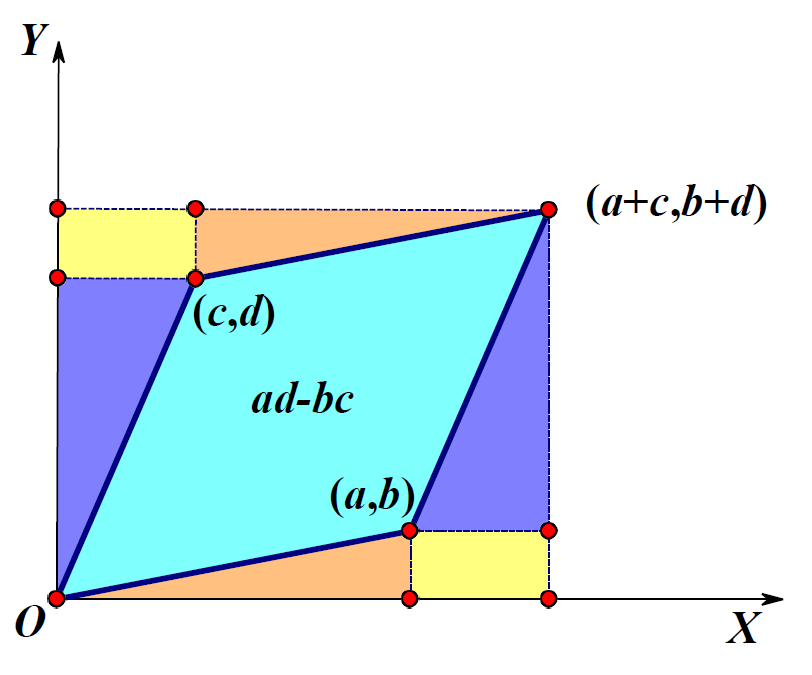（长文预警，推荐看完）。在初学行列式时，只知道它是一个数，有着一些神奇的性质。对于行列式的计算，我们在学习时会接触到逆序数这个词，但是并不知道为什么会有这个逆序数。

## 行列式的几何意义\begin{aligned} S&= (a+c)(b+d)-ab-cd-2bc \\ &= ad-bc \\ &= \begin{vmatrix} a & c \\ b & d \end{vmatrix} \end{aligned}

### 行列式几何意义的证明

\begin{aligned} v_1 & =v_1 \\ v_2^{\perp} &= v_2 - c_{12} v_1 \\ v_3^{\perp} &= v_3 - c_{13} v_1-c_{23} v_2 \\ & \vdots \end{aligned}

$$UU^T=diag(||v_1||^2,\cdots,||v_n^\perp||^2)$$

$$|UU^T|=||v_1||^2\cdots ||v_n^\perp||^2$$

### 非方阵的”行列式“

n维空间中m个列向量构成了一个$n\times m$的矩阵，记为$A$。我们知道行列式表示体积，但是只有方阵才有行列式，那应该如何处理呢？

$$A=QR,其中Q\in C^{n\times m},R\in C^{m\times m}$$

\begin{aligned} V &= |detR| \\ &= \sqrt{|det(R^TR)|} \\ &= \sqrt{|det((Q^TA)^TQ^TA)|} \\ &= \sqrt{|det(A^TA)|} \end{aligned}

### 楔积

1. （多重线性）：$\cdots \wedge(\lambda \vec{v}+\mu \vec{u}) \wedge \cdots=\lambda(\cdots \wedge \vec{v} \wedge \ldots)+\mu(\cdots \wedge \vec{u} \wedge \ldots)$
2. （反对称性）：$\cdots \wedge \vec{v} \wedge \cdots \wedge \vec{u} \wedge \cdots=(-1) \cdots \wedge \vec{u} \wedge \cdots \wedge \vec{v} \wedge \ldots$

\begin{aligned} \mathbf{a} \wedge \mathbf{b}&=\left(a_1 \mathbf{e}_1+a_2 \mathbf{e}_2\right) \wedge\left(b_1 \mathbf{e}_1+b_2 \mathbf{e}_2\right) \\ &=a_1 b_1 \mathbf{e}_1 \wedge \mathbf{e}_1+a_1 b_2 \mathbf{e}_1 \wedge \mathbf{e}_2+a_2 b_1 \mathbf{e}_2 \wedge \mathbf{e}_1+a_2 b_2 \mathbf{e}_2 \wedge \mathbf{e}_2 \\ &= (a_1b_2-a_2b_1)\mathbf{e}_1 \wedge \mathbf{e}_2 \end{aligned}

$$|A|=\frac{\mathbf{a}\wedge \mathbf{b}}{\mathbf{e}_1 \wedge \mathbf{e}_2}$$

$$|A|=||\mathbf{a} \wedge \mathbf{b}||$$

$$|A|=||a_1\wedge\cdots \wedge a_n||$$

$$|A|=\frac{\mathbf{a}\wedge \mathbf{b}}{\mathbf{e}_1 \wedge \mathbf{e}_2}$$

$$\operatorname{det} T=\left(T \overrightarrow{\mathrm{v}}_1 \wedge T \overrightarrow{\mathrm{v}}_2 \wedge \cdots \wedge T \overrightarrow{\mathrm{v}}_n\right) /\left(\overrightarrow{\mathrm{v}}_1 \wedge \overrightarrow{\mathrm{v}}_2 \wedge \cdots \wedge \overrightarrow{\mathrm{v}}_n\right)$$

$$V=||a_1\wedge \cdots \wedge a_m||$$

$$\vec{A} \cdot \vec{B}=\left(\vec{a}_1 \wedge \cdots \wedge \vec{a}_k\right) \cdot\left(\vec{b}_1 \wedge \cdots \wedge \vec{b}_k\right)=\left|\begin{array}{ccc} \vec{a}_1 \cdot \vec{b}_1 & \ldots & \vec{a}_1 \cdot \vec{b}_k \\ \vdots & \ddots & \vdots \\ \vec{a}_k \cdot \vec{b}_1 & \ldots & \vec{a}_k \cdot \vec{b}_k \end{array}\right|=\operatorname{det}\left(A^{\top} B\right)$$

## 行列式的代数意义

### 重线性函数

$$f\left(\cdots, a \mathbf{v}_1+b \mathbf{v}_2, \cdots\right)=a f\left(\cdots, \mathbf{v}_1, \cdots\right)+b f\left(\cdots, \mathbf{v}_2, \cdots\right)$$

### 对称重线性函数与交代重线性函数

$$f\left(\mathbf{v}_{\sigma(1)}, \cdots, \mathbf{v}_{\sigma(k)}\right)=f\left(\mathbf{v}_1, \cdots, \mathbf{v}_k\right)$$

$$f\left(\mathbf{v}_{\sigma(1)}, \cdots, \mathbf{v}_{\sigma (k) }\right)=\operatorname{sgn}\left(\sigma) f\left(\mathbf{v}_1, \cdots, \mathbf{v}_k\right)\right.$$

$$\operatorname{det}(\mathbf{A})=\operatorname{det}\left(\left[\begin{array}{llll} \mathbf{a}_1 & \mathbf{a}_2 & \cdots & \mathbf{a}_n \end{array}\right]\right)=\boldsymbol{A} \times_1 \mathbf{a}_1 \times_2 \mathbf{a}_2 \cdots \times_n \mathbf{a}_n$$

$$\operatorname{det}(\mathbf{A})=\left[\begin{array}{cc} 0 & 1 \\ -1 & 0 \end{array}\right] \times_1 \mathbf{a}_1 \times_2 \mathbf{a}_2=\left[\begin{array}{ll} a_{11} & a_{21} \end{array}\right]\left[\begin{array}{cc} 0 & 1 \\ -1 & 0 \end{array}\right]\left[\begin{array}{l} a_{12} \\ a_{22} \end{array}\right]$$

$$\boldsymbol{A}(:,:, 1)=\left[\begin{array}{ccc} 0 & 0 & 0 \\ 0 & 0 & 1 \\ 0 & -1 & 0 \end{array}\right], \boldsymbol{A}(:,:, 2)=\left[\begin{array}{ccc} 0 & 0 & -1 \\ 0 & 0 & 0 \\ 1 & 0 & 0 \end{array}\right], \boldsymbol{A}(:,:, 3)=\left[\begin{array}{ccc} 0 & 1 & 0 \\ -1 & 0 & 0 \\ 0 & 0 & 0 \end{array}\right]$$

$$\operatorname{det}(\mathbf{A})=\boldsymbol{A} \times_1 \mathbf{a}_1 \times_2 \mathbf{a}_2 \times_3 \mathbf{a}_3$$

$n$ 模积指的是张 量与矩阵的乘积，给定张量 $\mathcal{A} \in R^{I_1 \times I_2 \times \ldots \times I_N}$ ，矩阵 $U \in R^{J_n \times I_n}$ ，则张量 $\mathcal{A}$ 和矩阵 $U$ 的 $\mathrm{n}$ 模积表示为 $\mathcal{A}_{\times n} U$ ，是一个 $I_1 \times I_2 \times \ldots \times I_{n-1} J_n I_{n+1} \times \ldots \times I_N$ 的张量。并且张量 $\mathcal{A}_{\times n} U$ 中的元素具有如下计算公式
$$\left(\mathcal{A}_{\times n} U\right)_{i_1 i_2 \ldots i_{n-1} j_n i_{n+1} \ldots i_N}=\sum_{i_n=1}^{I_n} a_{i_1 i_2 \ldots i_{n-1} i_n i_{n+1} \ldots i_N} u_{j_n i_n}$$

## 行列式的应用

### 行列式优化问题的求解

\begin{aligned} \mathbf{D}&=\frac{\partial \operatorname{det}\left(\mathbf{X X}^T\right)}{\partial \mathbf{X}} \\ & =2 \operatorname{det}\left(\mathbf{X} \mathbf{X}^T\right)\left(\mathbf{X} \mathbf{X}^T\right)^{-1} \mathbf{X} \end{aligned}

\begin{aligned} & V_m=\frac{1}{(m-1) !} V_{m-1} h \\ & d V_m=\frac{1}{(m-1) !}\left(\left(d V_{m-1}\right) h+V_{m-1} d h\right) \\ & d V_m=\frac{1}{(m-1) !} V_{m-1} d h \\ & V_{m-1}=(m-1) \frac{d V_m}{d h} \end{aligned}

\begin{aligned} \mathbf{D D}^{\mathrm{T}}&=2 \operatorname{det}\left(\mathbf{X X}^{\mathrm{T}}\right)\left(\mathbf{X X}^{\mathrm{T}}\right)^{-1} \mathbf{X}\left(2 \operatorname{det}\left(\mathbf{X X}^{\mathrm{T}}\right)\left(\mathbf{X X}^{\mathrm{T}}\right)^{-1} \mathbf{X}\right)^{\mathrm{T}} \\ & =4 \operatorname{det}\left(\mathbf{X X}^{\mathrm{T}}\right)^2\left(\mathbf{X X}^{\mathrm{T}}\right)^{-1} \mathbf{X} \mathbf{X}^{\mathrm{T}}\left(\mathbf{X X}^{\mathrm{T}}\right)^{-1}=c\left(\mathbf{X X}^{\mathrm{T}}\right)^{-1} \end{aligned}

$$\left[\begin{array}{ll} \mathbf{A} & \mathbf{u} \\ \mathbf{u}^T & d \end{array}\right]^{-1}=\left[\begin{array}{cc} \mathbf{A}^{-1}+\mathbf{A}^{-1} \mathbf{u}\left(d-\mathbf{u}^T \mathbf{A}^{-1} \mathbf{u}\right)^{-1} \mathbf{u}^T \mathbf{A}^{-1} & -\mathbf{A}^{-1} \mathbf{u}\left(d-\mathbf{u}^T \mathbf{A}^{-1} \mathbf{u}\right)^{-1} \\ -\left(d-\mathbf{u}^T \mathbf{A}^{-1} \mathbf{u}\right)^{-1} \mathbf{u}^T \mathbf{A}^{-1} & \left(d-\mathbf{u}^T \mathbf{A}^{-1} \mathbf{u}\right)^{-1} \end{array}\right]$$

## 评论

1.雪宝
9月前
2023-3-20 23:11:06

感谢博主精彩分享，让学过矩阵线性代数的我都又眼前一亮！证明步骤清晰有条理，重温之余又get到了我之前没了解到的地方，（＞ｙ＜）再次感谢雨佬精心总结呀～～本雪宝悟辽

•博主
雪宝
9月前
2023-3-20 23:13:45

?

## 发送评论编辑评论

|´・ω・)ノ
ヾ(≧∇≦*)ゝ
(☆ω☆)
（╯‵□′）╯︵┴─┴
￣﹃￣
(/ω＼)
∠( ᐛ 」∠)＿
(๑•̀ㅁ•́ฅ)
→_→
୧(๑•̀⌄•́๑)૭
٩(ˊᗜˋ*)و
(ノ°ο°)ノ
(´இ皿இ｀)
⌇●﹏●⌇
(ฅ´ωฅ)
(╯°A°)╯︵○○○
φ(￣∇￣o)
ヾ(´･ ･｀｡)ノ"
( ง ᵒ̌皿ᵒ̌)ง⁼³₌₃
(ó﹏ò｡)
Σ(っ °Д °;)っ
( ,,´･ω･)ﾉ"(´っω･｀｡)
╮(╯▽╰)╭
o(*////▽////*)q
＞﹏＜
( ๑´•ω•) "(ㆆᴗㆆ)
( ゜- ゜)つロ
_(:з」∠)_
（⌒▽⌒）
（￣▽￣）
(=・ω・=)
(*°▽°*)八(*°▽°*)♪
✿ヽ(°▽°)ノ✿
(¦3【▓▓】
눈_눈
(ಡωಡ)
_(≧∇≦」∠)_
━━━∑(ﾟ□ﾟ*川━
(｀・ω・´)
(￣3￣)
✧(≖ ◡ ≖✿)
(･∀･)
(〜￣△￣)〜
→_→
(°∀°)ﾉ
╮(￣▽￣)╭
( ´_ゝ｀)
←_←
(;¬_¬)
(ﾟДﾟ≡ﾟдﾟ)!?
( ´･･)ﾉ(._.)
Σ(ﾟдﾟ;)
Σ( ￣□￣||)<
(´；ω；)
（/TДT)/
(^・ω・^)
(｡･ω･｡)
(●￣(ｴ)￣●)
ε=ε=(ノ≧∇≦)ノ
(´･_･)
(-_-#)
（￣へ￣）
(￣ε(#￣) Σ
(╯°口°)╯(┴—┴
ヽ(Д´)ﾉ
("▔□▔)/
(º﹃º )
(๑>؂<๑）
｡ﾟ(ﾟ´Д｀)ﾟ｡
(∂ω∂)
(┯_┯)
(・ω< )★
( ๑ˊ•̥▵•)੭₎₎
¥ㄟ(´･ᴗ･)ノ¥
Σ_(꒪ཀ꒪」∠)_
٩(๛ ˘ ³˘)۶❤
(๑‾᷅^‾᷅๑)

Emoji

tv_小电视